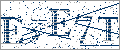>>

 Math >> Arithmatic >> Mathematics >> Area
Login or Register   Math - Perimeter of polygons and circle
 New User RegisterLogin Member: Email: Password:
 Site SearchAdvance Search

Other animationsMath - Area and PerimeterAnimation product List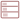Row format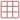Grid formatList formatOverview(For age - group : 11 - 16 )

Fully animated ‘Perimeter of polygons and circle ’ covers the concept using real life examples. This will help you to understand and calculate the perimeter of polygons and circles.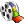Product - Animation
 Size/Time Subscribe Preview / Trailer Size (KB) 8644 US\$    1.50 Rs. 108.00  Check price in your currency Subscription Days = 30 Watch a preview (opens in separate window) Time (hr:min:sec) 0:50:0 Add To Cart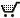Preview Unavailable

 This animation has audio.Category : Math Perimeter Type : Animation with sound Animation Type : Regular Total animation length: 50 minutes Watch combined previewThe animation covers perimeter of : Rectangle Square Parallelogram Rhombus Trapezium Triangle Circle and also sections on Formula Glossary Quiz section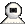Snapshots
 Watch combined previewWatch various movie clips related to Area and Perimeter. These are 1) Area and Perimeter 2) Area of Polygon and Circles 3) Perimeter of Polygon and Circles 4) Quiz on Area and Perimeter. Click on the above thumbnails to go to the movie.Perimeter is explained through real life examples and situations, the perimeter of polygons and circle is clearly explained through examples.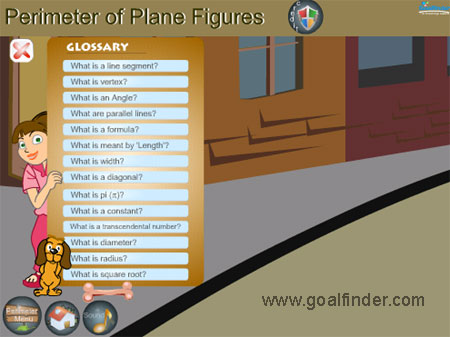An animated glossary is supported by examples for better understanding.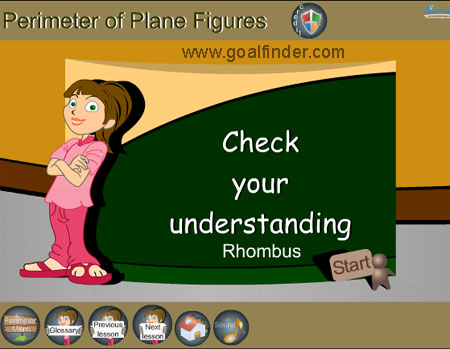Quiz or check your understanding is provided after each example section.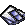Details of the animation/ movie /software

The animation starts by briefly explaining the concept of area and perimeter through an example of a farmer's field. Concept of a polygon, its properties, vertices, edges and body is then explained.

Perimeter section has situations and example for establishing the perimeter of various polygons, these firstly give the properties of each polygon and circle, then introduces an example of calculation followed by a quiz "Check your understanding" section, where each question has a detailed solution and there is no time limit or marking. The polygons covered in perimeters are

• Rectangle
• Square
• Parallelogram
• Rhombus
• Trapezium
• Triangle
• Circle

Glossary explains concepts of line segment, length, width, diagonal, diameter, radius etc. using definitions and examples. Following are covered

• Line Segment
• Angle
• Vertex
• Parallel Lines
• Formula
• Length, Width
• Diagonal
• Pi
• Constant
• Tanscendental Number
• Square Root

Formula section contains the summary of all the formula pertaining to polygon and circle.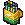Related Products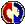Buyers Feedback:Q & A
 Q1: how much does all animation of maths cost Nilgün 3/1/2014 Ans: Q2: my dog namw oneshabennett 23/4/2013 Ans:Give your comments